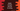# Kotlin program to convert one list to string## Introduction :

Converting one list to string in Kotlin is easy. Kotlin provides one method to do that easily. It is called joinToString. In this post, I will show you how we can convert one list to string in Kotlin using joinToString with examples.

### Definition :

This method is defined as below :

``````fun  Iterable.joinToString(
separator: CharSequence = ", ",
prefix: CharSequence = "",
postfix: CharSequence = "",
limit: Int = -1,
truncated: CharSequence = "...",
transform: ((T) -> CharSequence)? = null
): String``````

Here, separator : This is the element separator used to separate all elements. prefix : This is prefix of the final string postfix : This is the postfix of the final string limit : Number of first elements to append in the final string truncated : Truncated string transform : Transform operation

### Example of joinToString :

Let’s consider the below example :

``````fun main() {
val givenList = listOf(1, 2, 3, 4, 5)

println(givenList.joinToString())
println(givenList.joinToString(":", "{", "}", 4, "...etc") { (it * 2).toString() })
}``````

The first print method converted the list to string using joinToString and printed it. The second print method converted it using all parameters. It will print the below output :

``````1, 2, 3, 4, 5
{2:4:6:8:...etc}``````